DATA MINING
Desktop Survival Guide
by Graham Williams## Boxplot

A http://en.wikipedia.org/wiki/boxplotboxplot tukey:1977:eda (also known as a box-and-whisker plot) provides a graphical overview of how data is distributed over the number line. R's boxplot function displays a graphical representation of the textual summary of data. The skewness of the distribution of the data becomes clear.

A boxplot shows the http://en.wikipedia.org/wiki/medianmedian (the second http://en.wikipedia.org/wiki/quartilequartile or the 50th http://en.wikipedia.org/wiki/percentilepercentile) as the thicker line within the box (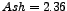). The top and bottom extents of the box (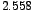and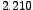respectively) identify the upper quartile (the third quartile or the 75th percentile) and the lower quartile (the first quartile and the 25th percentile). The extent of the box is known as the http://en.wikipedia.org/wiki/Interquartile_rangeinterquartile range (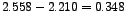). The dashed lines extend to the maximum and minimum data points that are no more than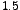times the interquartile range from the median. Outliers (points further thantimes the interquartile range from the median) are then individually plotted (at 3.23, 3.22, and 1.36). Our plot here adds faint horizontal lines to more easily read off the various values.

[width=0.65]rplot-wine-boxplot-single

 ```load("wine.Rdata") attach(wine) boxplot(Ash, xlab="Ash") abline(h=seq(1.4, 3.2, 0.1), col="lightgray", lty="dotted") ```

http://rattle.togaware.com/code/rplot-wine-boxplot-single.R

Subsections